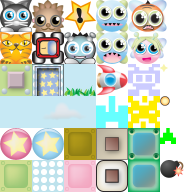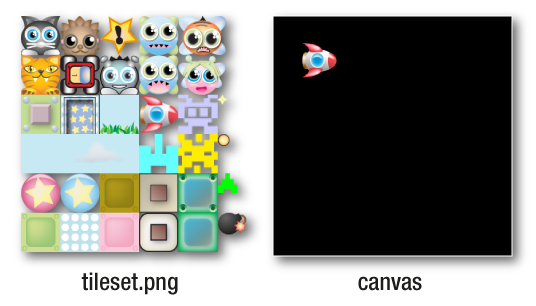# 从精灵图（雪碧图）中获取精灵loader  .add("images/tileset.png")  .load(setup);

function setup() {  //Create the tileset sprite from the texture  let texture = TextureCache["images/tileset.png"];  //Create a rectangle object that defines the position and  //size of the sub-image you want to extract from the texture  //(Rectangle is an alias for PIXI.Rectangle)  let rectangle = new Rectangle(192, 128, 64, 64);  //Tell the texture to use that rectangular section  texture.frame = rectangle;  //Create the sprite from the texture  let rocket = new Sprite(texture);  //Position the rocket sprite on the canvas  rocket.x = 32;  rocket.y = 32;  //Add the rocket to the stage  app.stage.addChild(rocket);  //Render the stage     renderer.render(stage);}

Pixi内置了一个通用的Rectangle对象 (PIXI.Rectangle)，他是一个用于定义矩形形状的通用对象。他需要一些参数，前两个参数定义了xy轴坐标位置，后两个参数定义了矩形的widthheight，下面是新建一个Rectangle对象的格式。

let rectangle = new PIXI.Rectangle(x, y, width, height);

let rectangle = new Rectangle(192, 128, 64, 64);texture.frame = rectangle;

let rocket = new Sprite(texture);